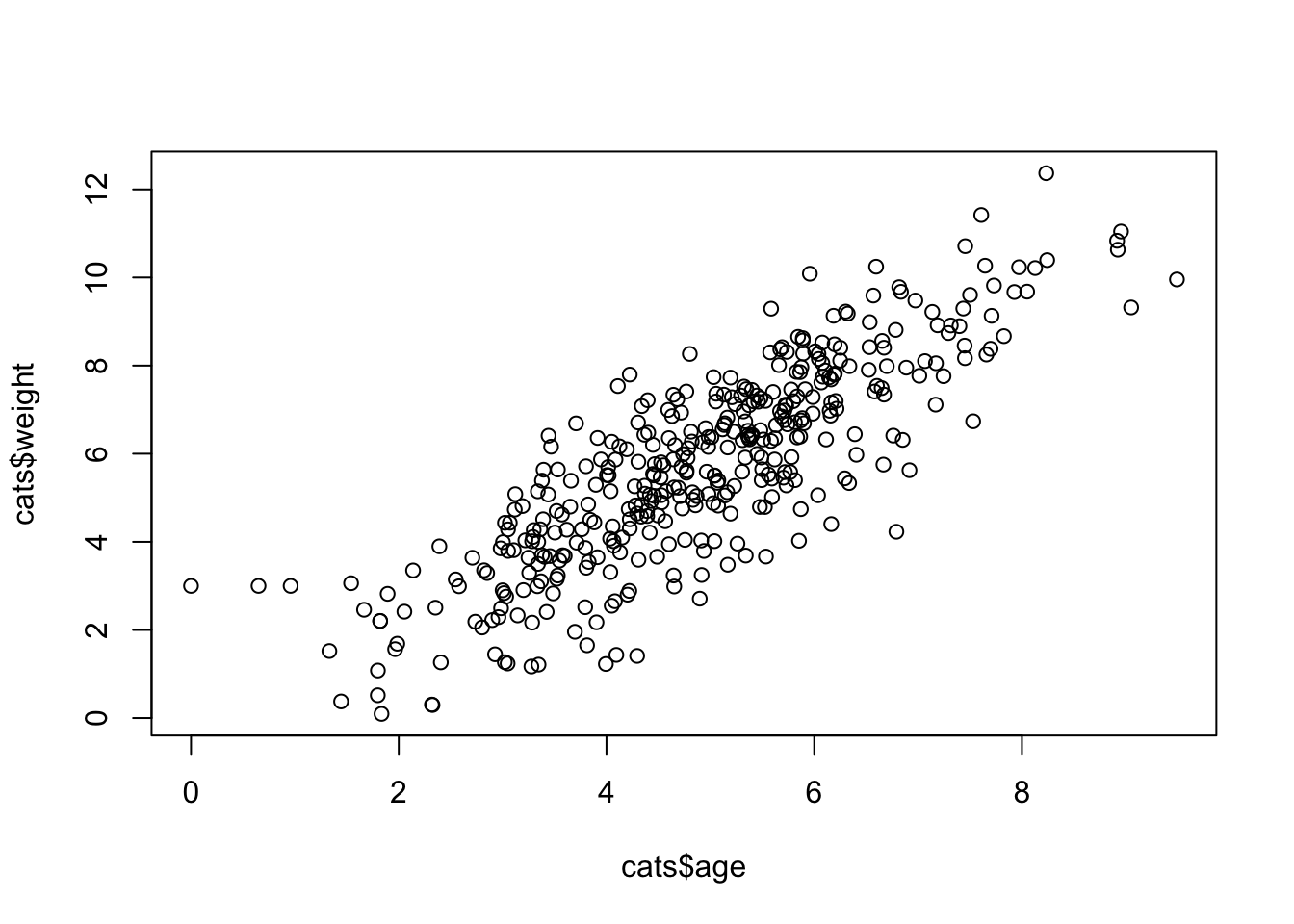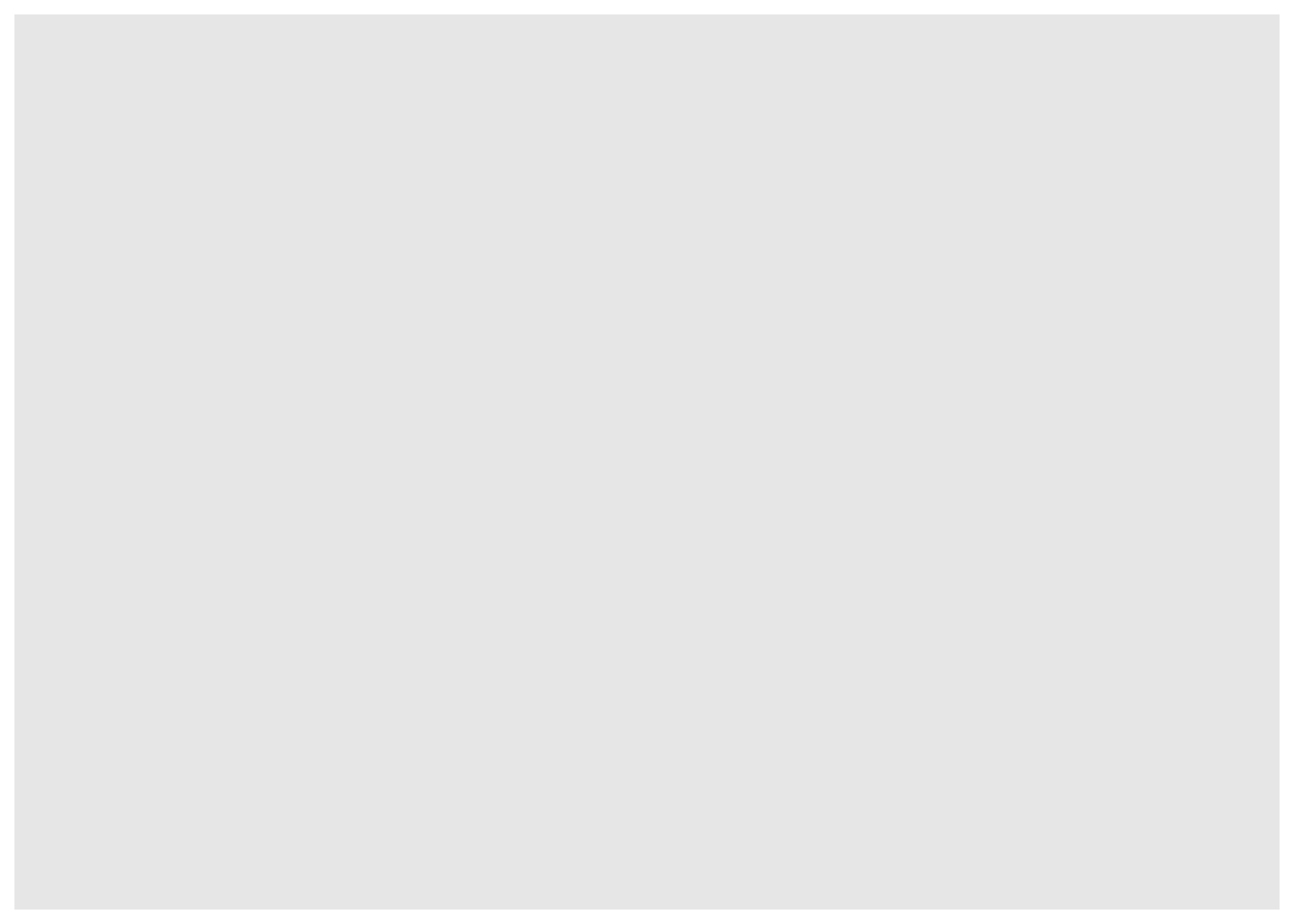## Learning Objectives

• Grammar of graphics concepts (geoms, aesthetics)
• Advanced plots (scales, facets, themes)
• Writing images (and other things) to file

# Basic plots in R

When we are working with large sets of numbers it can be useful to display that information graphically. R has a number of built-in tools for basic graph types such as hisotgrams, scatter plots, bar charts, boxplots and much more. We’ll test a few of these out here on the `cats` data.

``library(dplyr)``
``````##
## Attaching package: 'dplyr'``````
``````## The following objects are masked from 'package:stats':
##
##     filter, lag``````
``````## The following objects are masked from 'package:base':
##
##     intersect, setdiff, setequal, union``````
``cats <- read.csv("data/herding-cats.csv")``

## Scatterplot

Let’s start with a scatterplot. A scatter plot provides a graphical view of the relationship between two sets of numbers.

Let’s make a scatterplot of birth weight by mother’s age.

``plot(x = cats\$age, y = cats\$weight)``Each point represents a row in our dataset. The value on the x-axis is the mother’s age and the values on the y-axis correspond to the birth weight for the infant. For any plot you can customize many features of your graphs (fonts, colors, axes, titles) through graphic options

Exercises:

# Advanced figures (`ggplot2`)

More recently, R users have moved away from base graphic options and towards a plotting package called `ggplot2` that adds a lot of functionality to the basic plots seen above. The syntax is different but it’s extremely powerful and flexible. We can start by re-creating some of the above plots but using ggplot functions to get a feel for the syntax.

``install.packages("ggplot2")``

Load the `ggplot2` package.

``library(ggplot2)``

The `ggplot()` function is used to initialize the basic graph structure, then we add to it. The basic idea is that you specify different parts of the plot, and add them together using the + operator.

We will start with a blank plot and will find that you will get an error, because you need to add layers.

``ggplot(cats)``Geometric objects are the actual marks we put on a plot. Examples include:

• points (geom_point, for scatter plots, dot plots, etc)
• lines (geom_line, for time series, trend lines, etc)
• boxplot (geom_boxplot, for, well, boxplots!)

A plot must have at least one geom; there is no upper limit. You can add a geom to a plot using the `+` operator.

``````ggplot(cats) +
geom_point()``````

Each type of geom usually has a required set of aesthetics to be set, and usually accepts only a subset of all aesthetics –refer to the geom help pages to see what mappings each geom accepts. Aesthetic mappings are set with the `aes()` function.

Examples include:

• position (i.e., on the x and y axes)
• color (“outside” color)
• fill (“inside” color) shape (of points)
• linetype
• size

To start, we will add position for the x- and y-axis since geom_point requires mappings for x and y, all others are optional.

``````ggplot(cats) +
geom_point(aes(x = age, y = weight),
color = "red",
alpha = 0.5,
shape = 1,
size = 3)``````